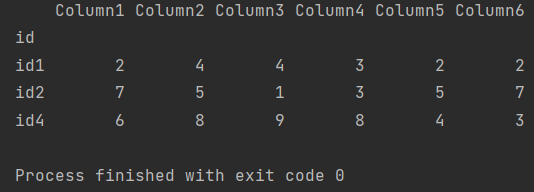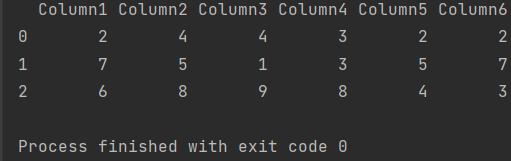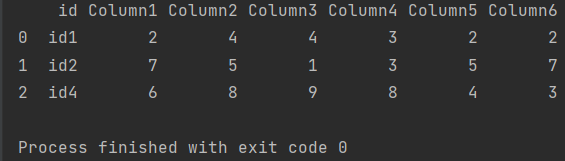Pandas How To Uncategorized How to remove index

# How to remove index

In this post you will learn how to remove index in Pandas.

When you use an index in your dataframe, it’s easier to work with. The condition, however, is that the index must not contain errors. If the index in your dataframe contains errors, it is better to remove such index.

I will show you how to delete an index using a simple dataframe example.

```import pandas as pd

my_df = pd.DataFrame({'id':['id1','id2','id4'],
'Column1': ['2', '7', '6'],
'Column2': ['4', '5', '8'],
'Column3': ['4', '1', '9'],
'Column4': ['3', '3', '8'],
'Column5': ['2', '5', '4'],
'Column6': ['2', '7', '3']},)

my_df.set_index('id',inplace=True)

print(my_df)
```

I created a dataframe and set the id column as the index.## How to remove index

At the last moment I noticed that the index is broken. I don’t like it, so I want to delete this index.

To remove the index in Pandas I used the reset_index function.

```import pandas as pd

my_df = pd.DataFrame({'id':['id1','id2','id4'],
'Column1': ['2', '7', '6'],
'Column2': ['4', '5', '8'],
'Column3': ['4', '1', '9'],
'Column4': ['3', '3', '8'],
'Column5': ['2', '5', '4'],
'Column6': ['2', '7', '3']},)

my_df.set_index('id',inplace=True)

my_df.reset_index(drop=True, inplace=True)

print(my_df)

```I removed the id column because I won’t need it. Another parameter I used is inplace to apply this change to my dataframe.

```import pandas as pd

my_df = pd.DataFrame({'id':['id1','id2','id4'],
'Column1': ['2', '7', '6'],
'Column2': ['4', '5', '8'],
'Column3': ['4', '1', '9'],
'Column4': ['3', '3', '8'],
'Column5': ['2', '5', '4'],
'Column6': ['2', '7', '3']},)

my_df.set_index('id',inplace=True)

my_df.reset_index(drop=False, inplace=True)

print(my_df)

```If I didn’t want to remove the id column, I should set the drop parameter to False.

## How to Create a 3D Pandas DataFrameHow to Create a 3D Pandas DataFrame

To create a 3D Pandas DataFrame, you can use a combination of the pd.Panel and pd.DataFrame objects. Here’s an example of how you can create a 3D DataFrame: import pandas

## How to join two dataframes on rowsHow to join two dataframes on rows

You can join two pandas DataFrames along the rows (i.e., concatenate them vertically) using the concat method in pandas. Here’s an example of how to concatenate two DataFrames vertically: